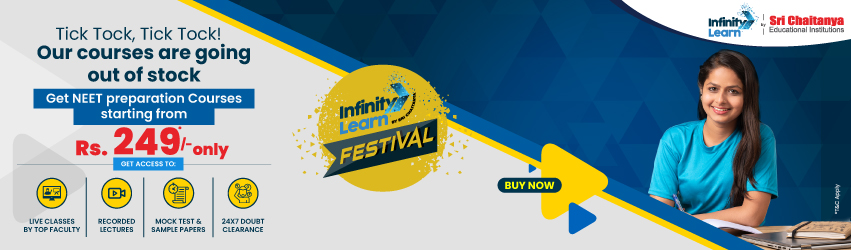NCERT Solutions for Class 11 Maths Chapter 16: Probability

# NCERT Solutions for Class 11 Maths Chapter 16: ProbabilityInfinity Learn NEET Festival
Infinity Learn IIT JEE Festival

Subject specialists have created NCERT Solutions for Class 11 Maths Chapter 16 Probability, including thorough solutions for reference. All of the questions from the textbook’s exercises are answered here. Students can use these answers to help them prepare for their exams. The NCERT Solutions for Class 11 provide useful solutions for improving conceptual knowledge.

The solutions are carefully solved using student-friendly terms while still adhering to the norms that must be followed when solving NCERT Solutions for Class 11. Practicing these answers can be incredibly advantageous not only in exams but also in helping Class 11 pupils perform well in upcoming competitive exams.

Need FREE NCERT/CBSE/IIT-JEE/NEET Study Material?

The approaches for answering have been given special consideration to stay on target while not deviating from the intended answer. Because time is so important in exams, excellent time management is essential for getting the best results when answering questions.

NCERT Solutions for Class 11 Maths Chapter 16 Probability

Do you need help with your Homework? Are you preparing for Exams? Study without Internet (Offline)
×
Chapter 16

NCERT Solutions for Class 11 Maths Chapter 16 Probability – Exercises

The chapter Probability discusses the general applications that we encounter on a regular basis. This chapter includes three tasks and a miscellaneous activity to help students fully grasp the fundamentals of probability. Chapter 16 Probability of NCERT Solutions for Class 11 Maths covers the following major subjects and subtopics:

16.1 – Introduction

This section includes a quick overview of the definition of probability and associated terminology. In basic terms, the probability is the ratio of favorable outcomes to the total number of outcomes. Experiment, sample space, occurrences, outcomes, and so on are linked concepts.

16.2 – Random Experiments

16.2.1 Outcomes and sample space

Students can easily understand the definition of a random experiment subject to the needed conditions and related terms by using examples such as tossing a coin and throwing a pair of dice.

16.3 -Event

16.3.1 Occurrence of an event

16.3.2 Types of events

16.3.3 Algebra of events

16.3.4 Mutually exclusive events

16.3.5 Exhaustive events

This topic has been discussed using numerous examples and experiments to thoroughly understand the terminology and types of occurrences. The intersection, union, difference, and complement of events are all explained by event algebra.

16.4 – Axiomatic Approach to Probability

16.4.1 Probability of an event

16.4.2 Probabilities of equally likely outcomes

16.4.3 Probability of the event ‘A or B.’

16.4.4 Probability of event ‘not A.’

The axiomatic approach is another technique for defining the likelihood of an event. This is nicely discussed in NCERT Maths Chapter 16 for Class 11 students. There are also numerous examples and practice problems to help you grasp the idea quickly.

Following are the concepts discussed in the NCERT Solutions of Infinity Learn:

• Sample space is a collection of all conceivable outcomes.
• The constituents of sample space are sample points.
• An event is a subset of the sample space.
• An empty set is an impossibility event.
• Sure thing: the entire sample area.
• ‘Not event’ or ‘complementary event’: The A′ or S – A set.
• The set A or B: the event A or B.
• A and B: The set A and B.
• The set A – B is made up of events A and not B.
• Mutually exclusive event: If A and B are mutually exclusive, then A and B are mutually exclusive.
• Events that are both exhausting and mutually exclusive.

Following are the Key Features of NCERT Solutions for Class 11 Maths Chapter 16 Probability

Infinity Learn’s solutions to Class 11 Maths NCERT questions assist students in reinforcing their fundamentals. They also believe in enhancing their self-esteem. Students can use all of the NCERT textbook solutions for Probability in their assignments, term – II exams, and competitive exams. In addition, the information is well-structured, so students will not be able to miss any steps in their preparation.

Frequently Asked Questions on NCERT Solutions for Class 11 Maths Chapter 16

1. What is Conditional Probability in Chapter 16 of NCERT Solutions for Class 11 Maths?
2. Explain a sample space covered in Chapter 16 of the NCERT Solutions for Class 11 Maths?
3. List out the major topics and subtopics covered in Chapter 16 of NCERT Solutions for Class 11 Maths?

1. Conditional Probability in Chapter 16 of NCERT Solutions for Class 11 Maths?

The term “conditional probability” refers to a situation where an event occurs only after confirmation that the first event has already occurred. In such circumstances, the two events are unrelated to one another. As a result, the chance of event A has no bearing on the probability of event B, making event A just the probability of event B. The answers PDF describes this concept in detail to help students do well in Class 11 second term exam.

2. Explain a sample space covered in Chapter 16 of the NCERT Solutions for Class 11 Maths?

A set contains all of the possible outcomes of a future event, such as selecting a card from many cards. It’s how you choose something from a set of samples and sample spaces. Each probability represents a small part of the sample space. As a result, the total number of choices will be one. Students can use the solutions, which are available in PDF format, to better understand this idea.

Join Infinity Learn Regular Class Programme!